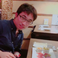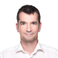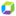cancel
Showing results for
Show  only  | Search instead for
Did you mean:

## How could I say if customer would ask me how reliable is "Root cause analysis"?Helper

Hi,

my customer (or trial user) have some ambiguous questions about Root cause analytics.
One of questions is: How reliable is "Root cause analysis"?

When I introduced dynatrace AI to them, they asked "What are the odds of correct root causes?".
I couldn't answer well, so I told them that I would explain the function of root cause again later after the investigation.

calculates the probability of individual incidents causing other incidents, applying an eigenvector centrality algorithm (the same ranking approach used by Google Search) to build a weighted graph of all related incidents to determine what issue has the highest statistical probability of being the root cause.

According this description, dynatrace calculates the probability of individual incidents causing other incidents as a weighted graph of all related incidents and determine what incident has the highest statistical probability of being the root cause.

I consider this probability can also be said as "the probability that the root cause is correct", which my our customer wants to know.

Is this understanding correct?
If so, how high is the probability to determine root cause? And is it relative value or absolute value?

I mean by "relative" that root causes are determined as a result of comparison of each probability of individual incidents causing other incidents.

At this case, for example, when the probability of incident "A" is 60% and that of B is 80%, B is higher than A, so B is determined as root cause.

I mean by "absolute" that root causes are determined as a result of comparison of the probability of each incident causing other incidents and some thresholds or baseline.

At this case, for example, when the threshold of probability for root cause is determined as 70% and incident "A" is 60% and that of B is 80%, B is over the threshold 70% so B is determined as root cause.

If my understanding is far from the fact, please tell me the correct understanding...

Regards,

Kohei Saito